# How to Solve HCF and LCM Questions Quickly – Educational Tips

0
401
views

HCF stands for Highest Common Factor whereas LCM stands for Least Common Multiple.

If you are facing problems in finding the LCM and HCF of two or more numbers or you don’t have time to solve a lot of questions, don’t worry.

In this guide, we will tell you how you can do it quickly with the help of online HCF and LCM calculators.

These calculators not only give the correct answers but also the complete solution of the problem under question.

But first of all, let’s discuss the concepts of HCF and LCM:

## Understanding the HCF

HCF of two or more numbers is the highest number that divides each of the numbers in the problem completely.

For example, in the case of three numbers, 14, 35, and 56, the highest number that divides each of these numbers is 7, which means 7 is the HCF of 14, 35, and 56.

This is because no number greater than 7 divides 14, 35, and 56 completely. That is why it is also called the Greatest Common Divisor.

The method to find the HCF is the following:

• Find the factors of all the numbers in the problem or express the numbers as a product of prime numbers
• Take the product of the prime numbers that are common among all the numbers in the question
• The resulting number is the highest common factor

### Example:

Q: Find the HCF of 36, 18, and 81.

Sol. Let’s find out the factors of the above numbers.

• 36 = 2 × 2 × (3 × 3)
• 18 = 2 × (3 × 3)
• 81 = (3 × 3) × 3 × 3

You can see 3 is two times a common factor among all the numbers in the question, so the multiplication of these two factors 3 × 3 is 9.

It means, 9 is the common factor of the above problem.

## How to find the HCF of two or more numbers quickly?

If you do not want to go through all the procedures of finding the HCF, you can skip it and find the assistance from an online HCF calculator.

There are plenty of tools available on the internet that help you to find HCF. All you have to do is to enter the numbers involved in the problem.

Let’s check some random tool on the internet to find our answer. We found a site named meracalculator where we were able to find both HCF and LCM of the given numbers.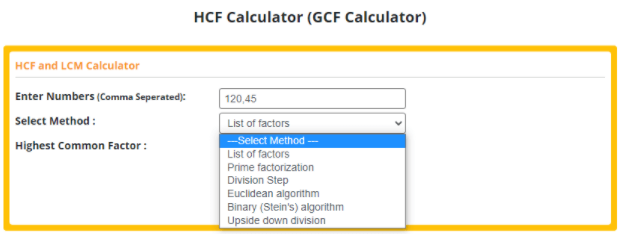The most amazing thing about this tool is that after entering the numbers, you can select the method to find the HCF.

After selecting the method, it gave us a complete solution with the correct answer.

We entered the numbers 36, 18, and 81 and select the “Prime factorization” method.

After clicking on the calculate button, we got the following solution: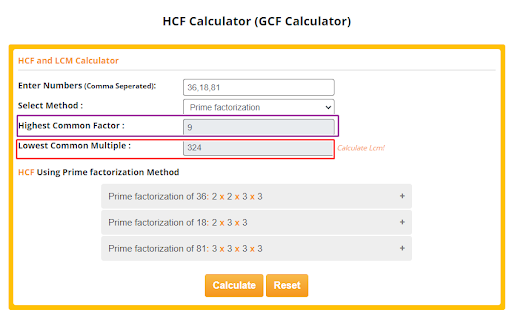It gave us the correct solution just by entering numbers, so it is a quick way to find HCF and LCM of two or more numbers.

Let’s select some other method and see how it reacts.

We entered 18 and 81 as input and select the “Division step” method.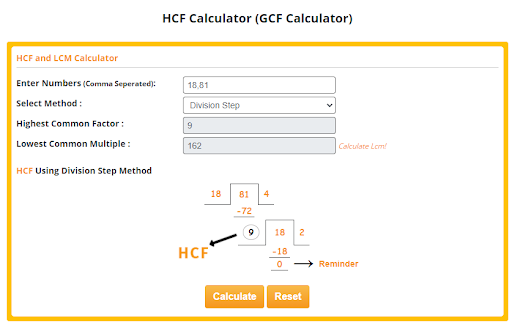It was quite interesting to see how it gave the complete solution of the division method as well.

So, if you want an instant solution with correct answers, we recommend you to use this tool.

You can select any of the following methods:

1. List of factors
2. Prime factorization
3. Division method
4. Euclidean algorithm
5. The binary algorithm or stein’s algorithm
6. Upside division

In this article, we tried only the Prime factorization and division method, however, if you want to explore other methods, you can try them as well.

## Understanding the LCM

The least common multiple of two or more numbers is the smallest number which is divisible by each of the numbers in the problem exactly.

For example, in the case of 18 and 24, 72 is the least common multiple.

The method of finding the LCM of two or more numbers is the following:

• Find the factors of all the numbers in the problem
• Take the product of prime numbers involved in each of the numbers after removing the recurrence of the common factors

### Example

Q: Find the LCM of 20 and 30.

Sol: Let’s find out the prime factors of both of the numbers in the problem.

• 20 = 2 × 2 × 5
• 30 = 2 × 3 × 5

You can see the common factors are 2 and 5 and it will be taken once in the finding the LCM. The other 2 and 3 are non-common. Our resulting multiplication is 2 × 2 × 3 × 5.

So, our resulting LCM will be 60.

## How to find LCM of two or more numbers quickly?

Now, it’s time to find the LCM of numbers using an online tool.

The most incredible thing about the tool we used in solving the HCF is that it also gives a separate calculator to find the LCM of the given numbers.

Let’s now try the LCM calculator of the meracalculator.com.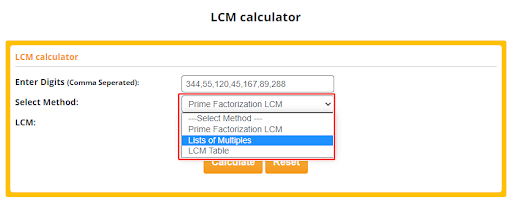LCM calculator also gave us three options as you can see in the picture above.

We entered the numbers and select the “List of Multiples” method and it gave us the following solution: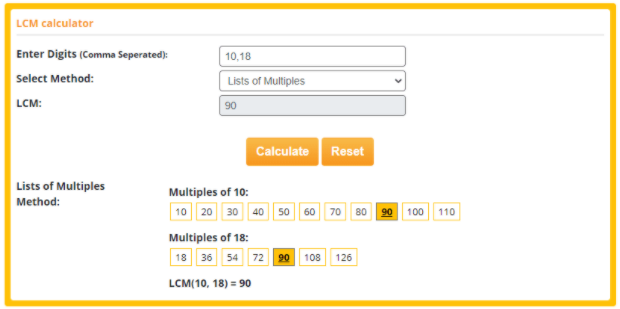LCM calculator also gave us the complete solution with an answer.

However, when we entered large numbers, it gave us the answer but not the solution.

It sent us the message “To many multiples to list here”.

So, we select the LCM table method and entered large numbers to see how it reacts in this situation.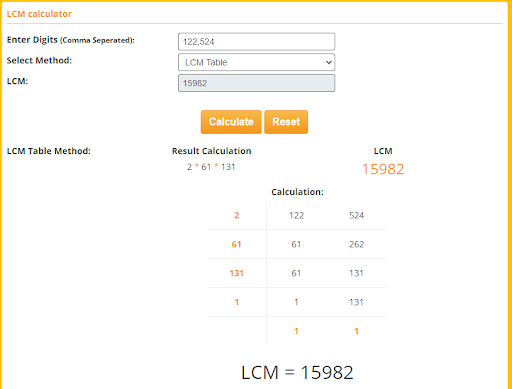The “LCM Table” method responded well regarding large numbers in this tool.

How useful it is that you get the answers and solution quickly at the same time and you save a lot of your time.

## Last words

You have seen how you can solve the problems of HCF and LCM instantly.

We recommend you use these tools if you do not have the time to solve all the questions in your assignment one by one. Use this technology and free yourself from worries.